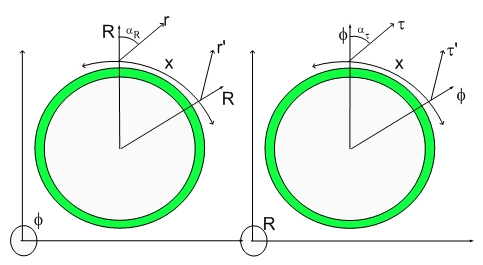# This is Music to My EarsThis is Music to My Ears

Up to now, Science has tried to run with a non-continuous paradigm where structured (Calaubi-Yao manifolds, compact manifolds or other infrastructure) space has been used to model the fragmented nature of matter, i.e. particles.

Even the question of dualism is incorrectly framed due to the lack of perception of the surrounding fourth spatial dimension. A particle is always a dilator (dilaton generator) which surfs the dilaton field, thus behaving like a dilaton itself. Of course, surfing is the result of the Quantum Lagrangian Principle from which I derived the Gravitation and Electromagnetism laws.

Particles are the basis for all the incorrect thinking we have around. A particle is steady, that is exist until it decays or doesn't as in the case of a proton or electron. This apparent steadiness gives rise to a bunch of steady qualities: mass, charge, momentum, spin...

If you realize that a particle is the center of a horospherical coordinate system in the 4D space. A horospherical coordinate system is the coordinate system centered at the dilaton wavefront. Under those circumstances, the 3D projection at the pseudo-quantized de Broglie radial expansion step is always a maximum deformation (contraction) of the metric. This means that pseudo time-quantization and being at the center of the dilaton wavefront creates an illusion of steadiness.

The Standard Model with Quarks, Gluons has been modeled mathematically by the inclusion of compact spaces (each modeling a given quantum degree of freedom) and the weak and strong forces.

This paradigm seems to me to be equivalent to the Rube Goldberg invention. I wouldn't touch it with a ten foot pole unless I had a very strong need.

If you think about a particle as a piece of something floating in space, you will never ask the following question: "What are the phase-matching conditions that the particle obey?"

This is exactly what I used to describe the meaning of material existence. Each particle in the Universe decayed from the Fundamental Universal Fluctuation, which I estimated as being a hyperspherical (4D) displacement volume with 15 kilometers radius.

The decay equation is irrelevant at this point, but one knows many other decay equations which bring into existence particles in a phase-matched condition. If you only see the three dimensional space, you miss that their existence is phase-matched with the now inexistent particle.

This is the basis for a new way to create energy through Coherent Nuclear Fusion. I will describe the process in detail in the Hypergeometrical Nuclear Fusion blog in the near future.

Now let's think why did I mentioned that "This is Music to My Ears"...:)

As you know, in my theory particles are dilators - coherences between 4D stationary deformation states- traveling at the speed of light along a radial direction.

There is a strong similarity with a source of sound. This should be obvious, but I will explain...:) Sound is a deformation of the density of air propagating at its natural speed. Dilatons are deformations of the metric (distance) propagating at its natural speed (the speed of light).

In that sense, it is clear that a particle (dilator) is a repeating chord. Thus all the Universe is a tremendous symphony or it is screaming God's name as it travels at the speed of light...:) as you wish...

In any event, the Universe is music to my ears...:)

Cheers

MP

PS- Next, I will introduce the other members of the Band: The Hyperons...:)

Currently unrated

### New Comment

required

required (not published)

optional

required